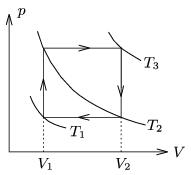Mathematical and Physical Journal
for High Schools
Issued by the MATFUND Foundation
 Already signed up? New to KöMaL?

#Problem P. 4241. (March 2010)

P. 4241. A sample of ideal gas is taken through the cyclic process shown in the figure.

a) What is the relation between T1, T2 and T3?

b) Express the efficiency of the heat engine which carry out this cyclic process in terms ofand.

c) In case of air between what values can this efficiency vary?(4 pont)

Deadline expired on April 12, 2010.

Sorry, the solution is available only in Hungarian. Google translation

Megoldás. $\displaystyle a)$ $\displaystyle T_2=\sqrt{T_1T_3},$

$\displaystyle b)$ $\displaystyle \eta=\frac{(\kappa-1)(x-1)}{1+\kappa x},$

$\displaystyle c)$ $\displaystyle 0<\eta<2/7$.

### Statistics:

 53 students sent a solution. 4 points: Balogh Gábor, Batki Bálint, Benyó Krisztián, Dénes Benjámin, Filep Gábor, Fonai Dániel, Janosov Milán, Kaposvári István, Nagy 111 Miklós, Pataki Bálint Ármin, Patartics Bálint, Varju 105 Tamás, Vuchetich Bálint, Zsámboki Richárd. 3 points: Béres Bertold, Bolgár Dániel, Czigány Máté Gábor, Farkas Martin, Fülöp András, Hartstein Máté, Hegedűs Csaba, Jéhn Zoltán, Kovács Attila, Kulcsár Flóra, Kungl Ákos Ferenc, Mayer Martin János, Molnár Alexandra, Pázmán Koppány, Pető János, Tamási Mátyás. 2 points: 4 students. 1 point: 8 students. 0 point: 6 students. Unfair, not evaluated: 5 solutionss.

Problems in Physics of KöMaL, March 2010